# THIS Keyword in Java with Example

## What is THIS Keyword in Java?

Keyword THIS is a reference variable in Java that refers to the current object.

The various usages of ‘THIS’ keyword in Java are as follows:Click here if the video is not accessible

### Understand ‘this’ keyword with an example.Let’s compile and run the code

Our expected output for A and B should be initialized to the values 2 and 3 respectively.

But the value is 0, Why? Let investigate.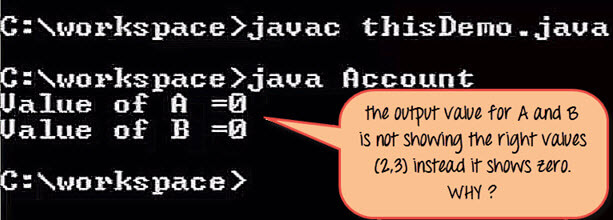In the method Set data, the arguments are declared as a and b, while the instance variables are also named as a and b.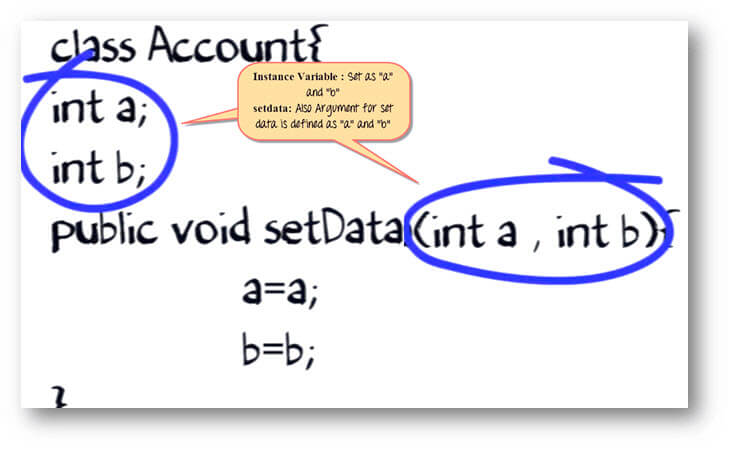During execution, the compiler is confused. Whether “a” on the left side of the assigned operator is the instance variable or the local variable. Hence, it does not set the value of ‘a’ when the method set data is called.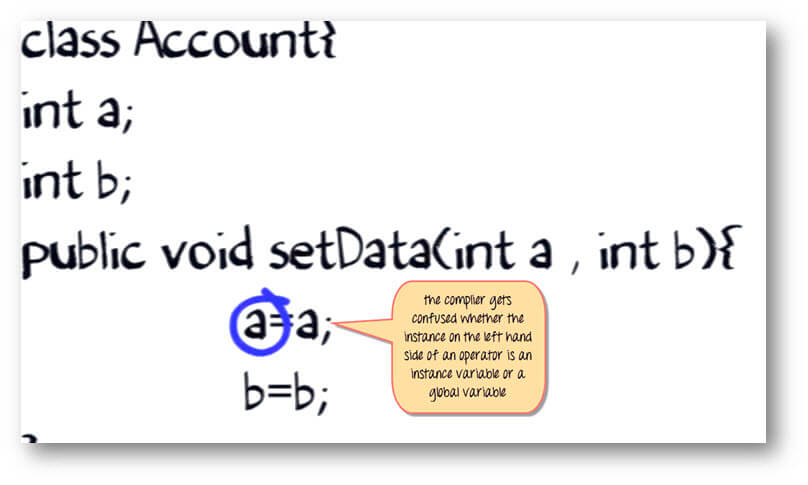The solution is the “THIS” keyword

Append both ‘a’ and ‘b’ with the “this” keyword followed by a dot (.) operator.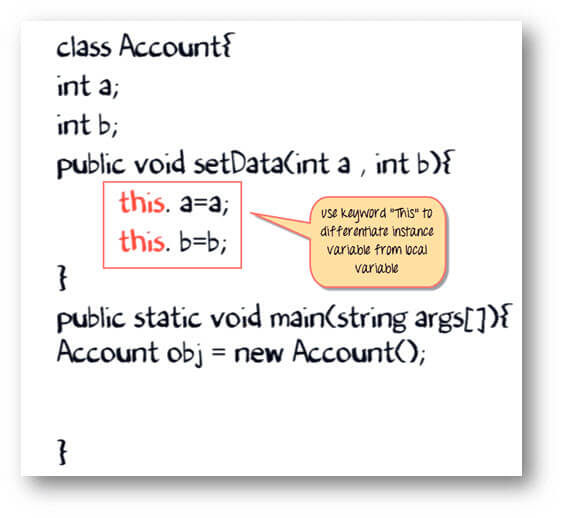The variables are initialized correctly, and the expected output is shown.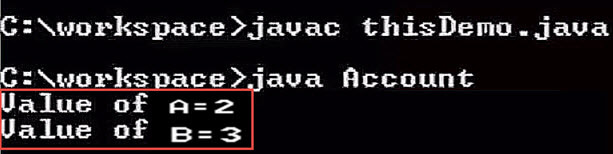Suppose you are smart enough to choose different names for your instance variable and methods arguments.But this time around, you create two objects of the class, each calling the set data method.

How the compiler will determine whether it is supposed to work on instance variable of object 1 or object 2.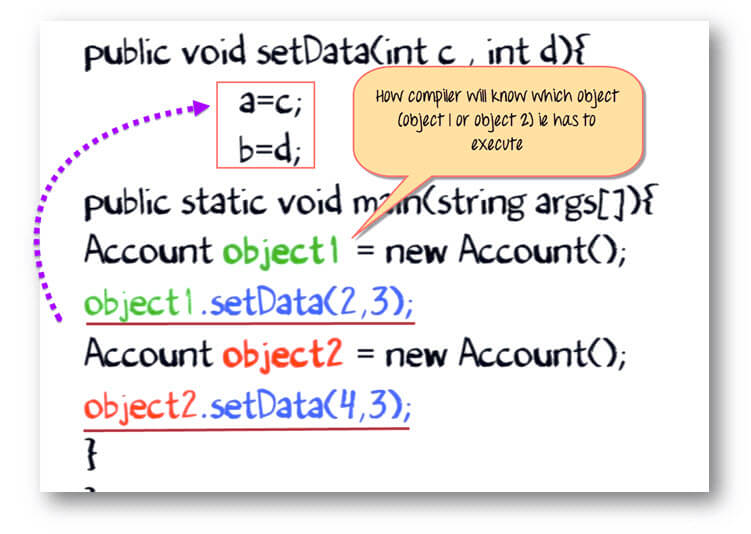Well, the compiler implicitly appends the instance variable with “THIS” keyword (image below).Such that when object 1 is calling the set data method, an instance variable is appended by its reference variable.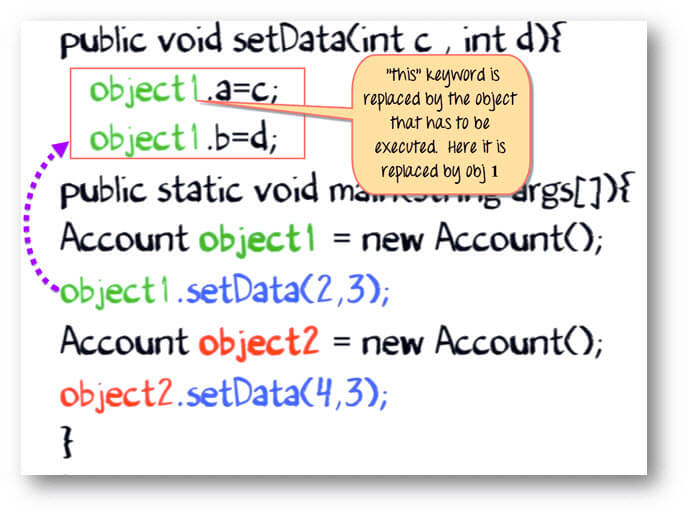While object 2 is calling the set data method, an instance variable of object 2 is modified.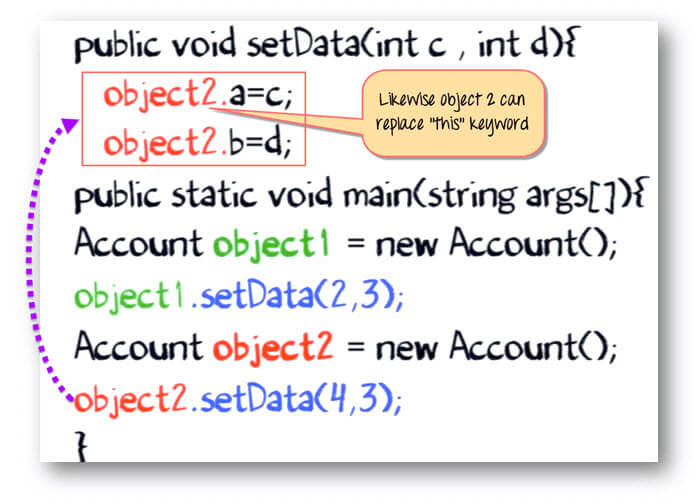This process is taken care by the compiler itself. You don’t have to append ‘this’ keyword explicitly unless there is an exceptional situation as in our example.

Example: To learn the use “this” keyword

Step 1) Copy the following code into a notepad.#### Top Courses